# NCERT Solutions For Class 9 Math Chapter – 6 Exercise – 6.1NCERT Solutions For Class 9 Math Chapter – 6 Exercise – 6.1

#### Experts (adsbygoogle = window.adsbygoogle || []).push({}); at Sunstarup  have created the NCERT Solutions after extensive search on each topic Students can refer to this study study material to boost their confidence and attempt the second term exam smartly. The concepts are explained with steps, shortcuts to remember formula , tips and tricks to solve the numerical problems wisely and quickly.

Q1.  In Fig. 6.13, lines AB and CD intersect at O. If AOC +BOE = 70° and BOD = 40°, find BOE and reflex COE.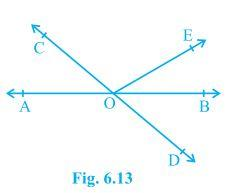Solution:

From the diagram, we have

(∠AOC +∠BOE +∠COE) and (∠COE +∠BOD +∠BOE) forms a straight line.

So, ∠AOC+∠BOE +∠COE = ∠COE +∠BOD+∠BOE = 180°

Now, by putting the values of ∠AOC + ∠BOE = 70° and ∠BOD = 40° we get

∠COE = 110° and ∠BOE = 30°

So, reflex ∠COE = 360o – 110o = 250o

1. In Fig. 6.14, lines XY and MN intersect at O. If POY = 90° and a : b = 2 : 3, find c.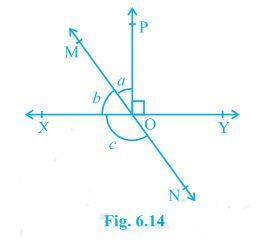Solution:

We know that the sum of linear pair are always equal to 180°

So,

POY +a +b = 180°

Putting the value of POY = 90° (as given in the question) we get,

a+b = 90°

Now, it is given that a : b = 2 : 3 so,

Let a be 2x and b be 3x

∴ 2x+3x = 90°

Solving this we get

5x = 90°

So, x = 18°

∴ a = 2×18° = 36°

Similarly, b can be calculated and the value will be

b = 3×18° = 54°

From the diagram, b+c also forms a straight angle so,

b+c = 180°

c+54° = 180°

∴ c = 126°

1. In Fig. 6.15, PQR = PRQ, then prove that PQS = PRT.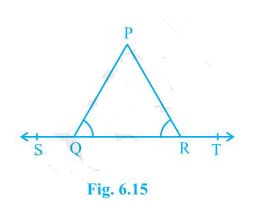Solution:

Since ST is a straight line so,

PQS+PQR = 180° (linear pair) and

PRT+PRQ = 180° (linear pair)

Now, PQS + PQR = PRT+PRQ = 180°

Since PQR =PRQ (as given in the question)

PQS = PRT. (Hence proved)

Q4.  In fig 6.16 If x + y = w + z then prove that AOB is a line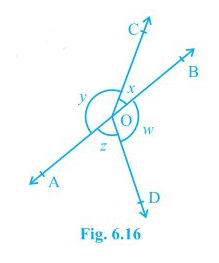Solution:-

X + y  = 1800

X + y + w + z = 3600

X+y = w + z

So , (x+y) + (x+y) = 3600

2(x+y) = 3600

(x+y) = 1800 (Hence proved)

Q5. In Fig 6.17 POQ is a line Ray OR is perpendicular to line PQ , OS is another ray lying between rays OP and OR. Prove that ROS = 1/2(QOS – POS).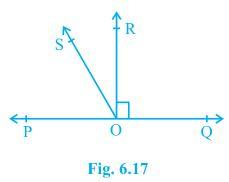Solution:-

POQ = 1800

POS + ROS + ROQ = 1800 – 900 (Since POR = ROQ = 900)

POS + ROS = 900

QOS = ROQ + ROS

QOS = 900 + ROS

QOS – ROS = 900

POS + ROS = 900 and  QOS – ROS = 900 we get

POS + ROS = QOS – ROS

2ROS + POS = QOS

Or , ROS = 1/2 (QOS – POS) (Hence proved).

Q6. It is given that XYZ = 640 and XY is produced to point P. Draw a figure from the given information If ray YQ bisects ZYP , find XYQ and reflex QYP.

Solution:-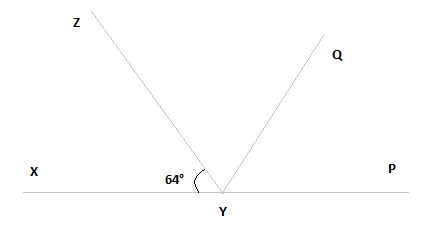Here , XP is a straight line

So , XYZ + ZYP = 1800

Putting the value of XYZ =  640 we get ,

640 + ZYP = 1800

ZYP = 1160

YQ bisects ZYP ,

ZYQ = QYP

ZYP = 2ZYQ

ZYQ = QYP = 580

XYQ = XYZ + ZYQ

By putting the value of XYZ = 640 and ZYQ = 580 we get

XYQ = 640 + 580

XYQ = 1220

QYP = 1800 + XYQ

XYQ = 1220

QYP = 1800 + 1220

QYP = 3020